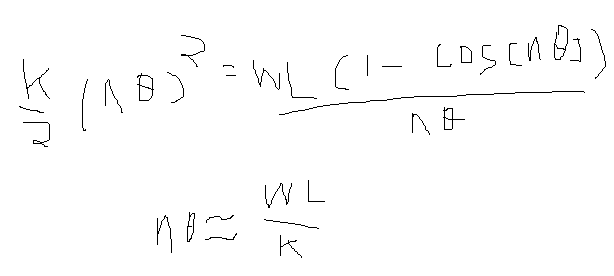# Beam Bending 2

So let’s assume k’ in the original equation is replaced with kn, with k a constant.

Then the equation becomes (as n tends to infinity)The position of the Mth hinge, as n tends to infinity, isThis is a parametric equation in M-theta (which ranges from zero to n-theta), but it turns out M can be eliminated symbolically, giving(Note that this is an ellipse; however, it is not even a full quarter of the ellipse, as x is generally not allowed to become as large as A)

One assumption we made in getting to this point was an assumption that the angles on all the hinges were equal, which could be considered rather goofy, at least without further justification / exploration.  Maybe we’ll look into that next time.